Publicité

# Differential Equations

26 Mar 2023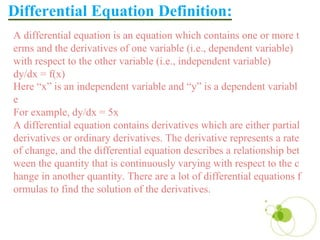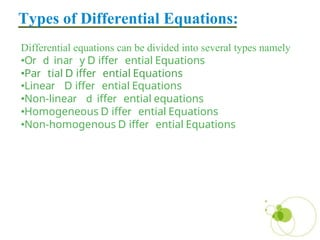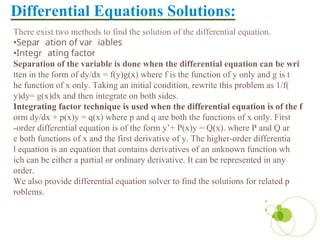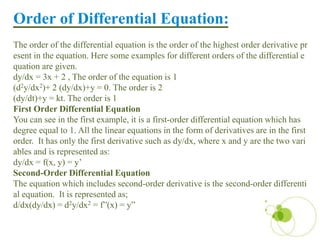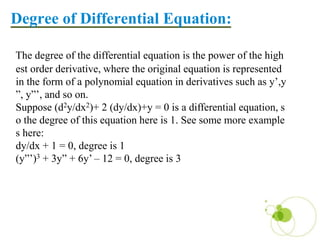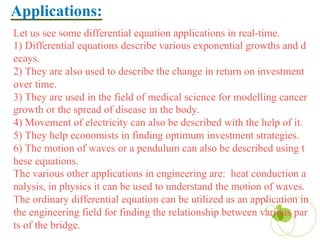1 sur 8
Publicité

### Differential Equations

1. Integral Calculus & Differential Equations TAE-II Amritansh Manthapurwar DIFFERENTIAL EQUATIONS E-09
2. A differential equation is an equation which contains one or more t erms and the derivatives of one variable (i.e., dependent variable) with respect to the other variable (i.e., independent variable) dy/dx = f(x) Here “x” is an independent variable and “y” is a dependent variabl e For example, dy/dx = 5x A differential equation contains derivatives which are either partial derivatives or ordinary derivatives. The derivative represents a rate of change, and the differential equation describes a relationship bet ween the quantity that is continuously varying with respect to the c hange in another quantity. There are a lot of differential equations f ormulas to find the solution of the derivatives. Differential Equation Definition:
3. Differential equations can be divided into several types namely •O ina y iffe ential Equations •Pa tial iffe ential Equations •Linea iffe ential Equations •Non-linea iffe ential equations •Homogeneous iffe ential Equations •Non-homogenous iffe ential Equations Types of Differential Equations:
4. There exist two methods to find the solution of the differential equation. •Sepa ation of va iables •Integ ating facto Separation of the variable is done when the differential equation can be wri tten in the form of dy/dx = f(y)g(x) where f is the function of y only and g is t he function of x only. Taking an initial condition, rewrite this problem as 1/f( y)dy= g(x)dx and then integrate on both sides. Integrating factor technique is used when the differential equation is of the f orm dy/dx + p(x)y = q(x) where p and q are both the functions of x only. First -order differential equation is of the form y’+ P(x)y = Q(x). where P and Q ar e both functions of x and the first derivative of y. The higher-order differentia l equation is an equation that contains derivatives of an unknown function wh ich can be either a partial or ordinary derivative. It can be represented in any order. We also provide differential equation solver to find the solutions for related p roblems. Differential Equations Solutions:
5. esent in the equation. Here some examples for different orders of the differential e quation are given. dy/dx = 3x + 2 , The order of the equation is 1 (d2y/dx2)+ 2 (dy/dx)+y = 0. The order is 2 (dy/dt)+y = kt. The order is 1 First Order Differential Equation You can see in the first example, it is a first-order differential equation which has degree equal to 1. All the linear equations in the form of derivatives are in the first order. It has only the first derivative such as dy/dx, where x and y are the two vari ables and is represented as: dy/dx = f(x, y) = y’ Second-Order Differential Equation The equation which includes second-order derivative is the second-order differenti al equation. It is represented as; d/dx(dy/dx) = d2y/dx2 = f”(x) = y” The order of the differential equation is the order of the highest order derivative pr Order of Differential Equation:
6. est order derivative, where the original equation is represented in the form of a polynomial equation in derivatives such as y’,y ”, y”’, and so on. Suppose (d2y/dx2)+ 2 (dy/dx)+y = 0 is a differential equation, s o the degree of this equation here is 1. See some more example s here: dy/dx + 1 = 0, degree is 1 (y”’)3 + 3y” + 6y’ – 12 = 0, degree is 3 The degree of the differential equation is the power of the high Degree of Differential Equation:
7. Let us see some differential equation applications in real-time. 1) Differential equations describe various exponential growths and d ecays. 2) They are also used to describe the change in return on investment over time. 3) They are used in the field of medical science for modelling cancer growth or the spread of disease in the body. 4) Movement of electricity can also be described with the help of it. 5) They help economists in finding optimum investment strategies. 6) The motion of waves or a pendulum can also be described using t hese equations. The various other applications in engineering are: heat conduction a nalysis, in physics it can be used to understand the motion of waves. The ordinary differential equation can be utilized as an application in the engineering field for finding the relationship between various par ts of the bridge. Applications:
8. THANK YOU THANK YOU
Publicité